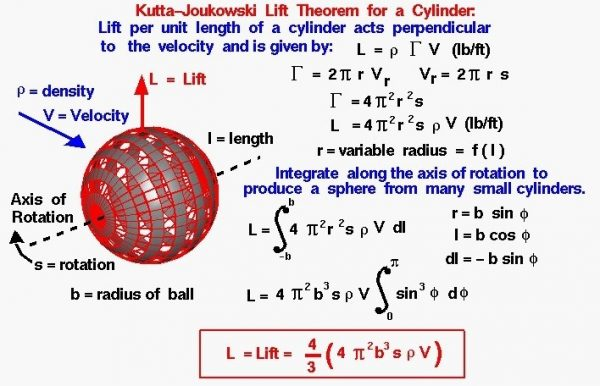# Ideal Lift of a Spinning BallAll that is necessary to create lift is to turn a flow of air. The airfoil of a wing turns a flow, and so does a rotating cylinder. A spinning ball also turns a flow and generates an aerodynamic lift force.

## Aerodynamic Lift Force

The details of how a spinning ball creates lift are fairly complex. Next to any surface, the molecules of the air stick to the surface, as discussed in the properties of air slide. This thin layer of molecules entrains or pulls the surrounding flow of air. For a spinning ball the external flow is pulled in the direction of the spin. If the ball is not translating, we have a spinning, vortex-like flow set up around the spinning ball, neglecting three-dimensional and viscous effects in the outer flow. If the ball is translating through the air at some velocity, then on one side of the ball the entrained flow opposes the free stream flow, while on the other side of the ball, the entrained and free stream flows are in the same direction. Adding the components of velocity for the entrained flow to the free stream flow, on one side of the ball the net velocity is less than free stream; while on the other, the net velocity is greater than free stream. The flow is then turned by the spinning ball, and a force is generated. Because of the change to the velocity field, the pressure field is also altered around the ball. The magnitude of the force can be computed by integrating the surface pressure times the area around the ball. The direction of the force is perpendicular (at a right angle) to the flow direction and perpendicular to the axis of rotation of the ball.

## Geometry

On the figure at the left, we show the geometry of the spinning ball. A ball of radius b rotates at speed s measured in revolutions per second. A black dashed line indicates the axis of rotation of the ball, and the ball rotates clock-wise, when viewed along the axis from the lower left. The ball has been sliced into a large number of grey-colored sections along the axis of rotation. The air with velocity V and density rho strikes the ball from the upper left. The resulting lift force L is perpendicular to the air velocity and the axis of rotation.

To determine the ideal lift force on the ball, we consider the spinning ball to be composed of an infinite number of very small, grey-colored, rotating cylinders. Adding up (integrating) the lift of all of the cylinders along the axis gives the ideal lift of the ball.

## Kutta-Joukouski Lift Theorem

The Kutta-Joukowski lift theorem for a single cylinder states the lift per unit length L is equal to the density rho of the air times the strength of the rotation Gamma times the velocity V of the air:

### L = rho * Gamma * V

The strength of rotation is directly related to the rotational speed of the cylinder. For a cylinder of radius r rotating at angular speed s, the surface of the cylinder moves at speed Vr given by:

### Vr = 2 * pi * r * s

Then the strength of rotation is equal to:

### Gamma = 2 * pi * r * Vr

Combining these equations:

### Gamma = 4 * pi^2 * r^2 * s

And substituting this value into the Kutta-Joukowski lift equation give:

### L = 4 * pi^2 * r^2 * s * rho * V

This is the lift per unit length for a single small cylinder and is measured in force per length (lbs/ft). The ball is composed of an infinite number of cylinders with the radius of the cylinders changing along the axis of rotation.

### r = f (l)

where l is a length measured along the axis of rotation. For a ball of radius b we have to integrate the lift per unit length along the axis of rotation from -b to +b. If we let S be the symbol for integration and dl be an increment of l:

### L = S (4 * pi^2 * r^2 * s * rho * V) dl

If we let phi be an angle from the center of the ball along the axis of rotation:

Then:

### L = 4 * pi^2 * b^3 * s * rho * V * S (sin (phi))^3 d(phi)

with the limits of integration going from zero to pi. Performing the integration:

## Analysis

Be particularly aware of the simplifying assumptions that have gone into this analysis. The type of flow field shown on this figure is called an ideal flow field. We have produced the ideal flow field by superimposing the flow field from an ideal vortex centered on the ball with a uniform free stream flow. There is no viscosity in this model, and therefore, no boundary layer on the ball, even though viscosity is the real origin of the circulating flow!

The equation given above describes the ideal lift force generated on a smooth, rotating ball. We can use this description as a first order, or preliminary, estimate of the lift on a baseball or a soccer ball. But in reality, the flow around a spinning baseball or soccer ball is very complex. Viscosity generates a boundary layer on the ball and the stitches used to hold the covering of the ball together stick up out of the boundary layer and disturb both the boundary layer and free stream flow. On the ball, the boundary layer transitions to turbulent flow which affects the amount of aerodynamic drag. The stitches are not symmetrically distributed around the ball. So the real flow around the ball is separated, unsteady, and not uniform. To account for these real-world effects that we have neglected in our ideal flow model, we define a lift coefficient. The lift coefficient is an experimentally determined factor that is multiplied times the ideal lift value to produce a real lift value. The ideal simplified model gives the first order effects and tells us the relative importance of the factors that affect the lift force on the ball, while all of the complex factors are modeled by the lift coefficient.